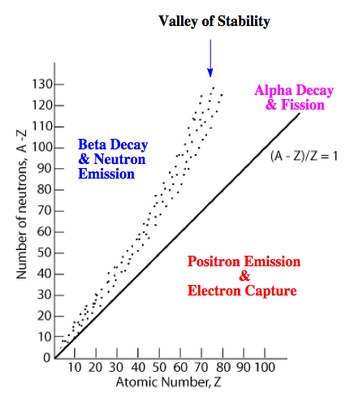## General Chemistry

Learn the toughest concepts covered in Chemistry with step-by-step video tutorials and practice problems by world-class tutors

21. Nuclear Chemistry

# Band of Stability

The Valley or Band of Stability represents the area where stable, non-radioactive isotopes exist based on their ratio of neutrons to protons.

Valley of Stability

Whenever the difference between neutrons to protons within a nucleus is significant enough an isotope is radioactive.

1
concept

## The Neutron to Proton Ratio3m
Play a video:

Neutrons act like the glue that keeps the nucleus together. The more neutrons present then the greater the attractive strong force, while the more protons then the greater the repulsive Coulombic force

2
concept

## Valley of Stability10m
Play a video:3
example

## Valley of Stability3m
Play a video:
So for this first example, it says determine that the following nuclei will undergo Alfa decay, beta decay or positron emission. So remember the way you approach this question is remember that if you do Alfa Decay, that usually happens if you're atomic mass, which is a is two hundred, um, am use or higher. And remember your atomic masses, the number they're giving you here would be three. So obviously it's not gonna do Alfa Decay. We're going to stay here. Beta decay, how it beta decay happened. That means you're above the valley of stability and then positron emission. We'd say that that would be below the valley of stability. So we know Alfa decay is not gonna happen because because our atomic mass is not two hundred or higher. So that's off the table. We have hydrogen three. So that means it's atomic masses. Three hydrogen has an atomic number of one. Now, hydrogen three also has another name. It's called Treaty. Um, so here we'll still put H, but usually we symbolize it with tea. What we're gonna do here is we have to figure out what the neutron to proton ratio is. So the atomic number is the number of protons, which is one. And then to find a number of neutrons, you just so track these two. So that's two. Now, remember, from the previous page, we said that Elham isotopes that have an atomic mass or actually atomic number Sorry, atomic number between one and twenty, their most stable when their ratio is equal toe one because that will put them right in the valley of stability. Here, our number is not one. It's too. That's a number that's higher than the number we want. So it would be above the valley of stability. And if you're above the valley of stability, that means you're undergo a beta decay. So we're gonna spit out an electron. Then we have to just make sure we balance the radioactive process here. So zero plus three would give me this three here and then minus one here, but we want a one at the end. So we have to put a two here that would give me helium. So we'd say that hydrogen three would undergo a beta decay in order to produce helium three. So that's the approach you take. First of all, does it have an atomic mass that is two hundred a. M. You or higher. If it does, it'll do Alfa Decay out of the three choices. Then, if it's not that, look to see what the neutron to proton ratio is. If it's above our racial that we want for the Valley of Stability, it'll do beta decay. If it's below it, it'll do. Positron emission. That's the approach you need to take. Now follow what we just did in this example to see if you can answer example to if you can, it's okay. Just come back and see how I approach this question to get the answer.
4
example

## Valley of Stability1m
Play a video:
So here we have Radan to 22. So right on symbol is RN and then to 22 means that that's its atomic mass. And then if you look on the periodic table, you'll see that Radan itself has an atomic number of 86. All right, so remember beta decays. If our our neutron to proton ratio is above the value of stability, positron emission is if we're below the valley of stability and then Alfa decay is the most common type of decay. If you're atomic, mass is 200 or higher here, our atomic mass is definitely 200 or higher. So this is gonna undergo in Alfa decay, which means that we would spit out in half a particle which is four over to. And here's our symbol for Alfa. We could also do four over to for helium because it has on the same exact atomic mass and atomic number plus All right, so we need the right side to add up to 2 22 like it is here, so to 20 to minus four would mean that the new element being created would have to be having atomic mass of to 18 here. It's 86. We already have two here, so this would have to be 84. When we look on the periodic table, that gives us Pio, which is polonium. So we'd say here that raid on 2 22 undergoes an Alfa decay in order to create polonium 2 18 by Alfa Decay. And again, we know it's Alfa Decay because of the atomic mass. As long as it's 200 a. M. You are higher. Alfa Decay is the most common type of radioactive decay that will take place. So two down and one left to go. Try to see if you can answer this question if you can, it's okay, come back and see how I approach it.
5
example

## Valley of Stability2m
Play a video: